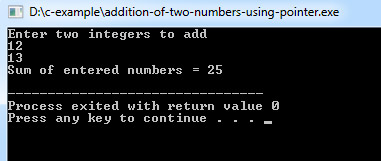## C Programming Examples Tutorial Index

This program performs addition of two numbers using pointers.

In this program I have used two integer variables x, y and two pointer variables p and q.

Firstly I have assign the addresses of x and y to p and q respectively and then assign the sum of x and y to variable sum. & is address of operator and * is value at address operator.

Example:

``````#include <stdio.h>

int main()
{
int first, second, *p, *q, sum;

scanf("%d%d", &first, &second);

p = &first;
q = &second;

sum = *p + *q;

printf("Sum of entered numbers = %d\n",sum);

return 0;
}``````

Program Output: